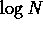Next: List of record numbers Up: Prime Numbers Previous: Algorithms to factor integer

## Primality Testing

The problem of primality testing and factorization are two distinct problems. If we concentrate on primality testing, we never need to know the actual factors. The only question to be answered is "is the number in question prime or composite."

Wilson's Theorem: The integer p is prime if and only if (p-1)! is congruent to -1 (mod p)

Since there is no known method for rapidly computing (N-1)! (mod N) in, say,steps, so Wilson's characterization of primes is of no practical value to the testing of the primality of N.

There are many different primality tests and they can be classified in at least three different ways:

1. Tests for numbers of special forms
versus
Tests for generic numbers
2. Tests with full justification
versus
Tests with justification based on conjectures
3. Deterministic tests
versus
Probabilistic or Monte Carlo tests

Miller's Test

In 1976, G. L. Miller proposed a primality test, which was justified using a generalized form of Riemann's hypothesis.

The APR Test

The primality test devised by L. M. Adleman, C. Pomerance and R. S. Rumely (1983), also known as the APR test, represents a breakthrough because:

1. It is applicable to arbitrary natural numbers N, without requiring the knowledge of factors of N - 1 or N + 1.
2. The running time t(N) is almost polynomial.
3. The test is justified rigorously, and for the first time ever in this domain, it is necessary to appeal to deep results in the theory of algebraic numbers; it involves calculations with roots of unity and the general reciprocity law for the power residue symbol.

The running time of the APR is at the present the world record for a deterministic primality test.

Soon afterwards, H. Cohen & A. K. Lenstra (1984) modified the APR test, making it more flexible, using Gauss sums in the proof (instead of the reciprocity law), and having the new test programmed for practical applications. It was the first primality test in existence that can routinely handle numbers of up 100 decimal digits, and it does so in about 45 seconds.

Monte Carlo methods

Ribenboim mentions three Monte Carlo tests, due to R. Baillie & Wagstaff, Jr. (1980), R. Solovay & V. Strassen (1977), and M. O. Rabin (1976, 1980).

Elliptic Curves Primality Proving, ECPP

ECPP stands for "Elliptic Curves and Primality Proving". The algorithm is described in:

```    A. O. L. Atkin and F. Morain
"Elliptic curves and primality proving"
To appear.```

It is a deterministic algorithm that gives a certificate of primality for numbers that can be as large as 10**1000 (one thousand).

References

``` A. O. L. Atkin and F. Morain
"Elliptic curves and primality proving"
To appear in Math. Comp.

%  Lieven Marchand <mal@bewoner.dma.be>

 F. Morain
"Courbes elliptiques et tests de primalite'"
The`se, Universite' de Lyon I, 1990.
Available at:
http://lix.polytechnique.fr/~morain/english-index.html```

This subsection is copyright (C) 1995. Harry J. Smith, HJSmith@ix.netcom.com.Next: List of record numbers Up: Prime Numbers Previous: Algorithms to factor integer

Alex Lopez-Ortiz
Mon Feb 23 16:26:48 EST 1998